Reach Us+44-1522-440391
Efficient Analysis of Photonic Crystal Slabs | OMICS International
Journal of Lasers, Optics & Photonics
All submissions of the EM system will be redirected to Online Manuscript Submission System. Authors are requested to submit articles directly to Online Manuscript Submission System of respective journal.

# Efficient Analysis of Photonic Crystal Slabs

Mohammad Hasan Aram and Sina Khorasani*

School of Electrical Engineering, Sharif University of Technology, Tehran, Iran

*Corresponding Author:
Sina Khorasani
School of Electrical Engineering
Sharif University of Technology, Tehran, Iran
Tel: +98-21-6616-4352
E-mail: [email protected]

Received Date: October 31, 2014; Accepted Date: December 01, 2014; Published Date: December 12, 2014

Citation: Aram MH, Khorasani S (2014) Efficient Analysis of Photonic Crystal Slabs. J Laser Opt Photonics 1:111. doi:10.4172/2469-410X.1000111

Copyright: © 2014 Aram MH, et al. This is an open-access article distributed under the terms of the Creative Commons Attribution License, which permits unrestricted use, distribution, and reproduction in any medium, provided the original author and source are credited.

Visit for more related articles at Journal of Lasers, Optics & Photonics

#### Abstract

In this paper we propose an accelerated plane wave expansion method (PWEM) for calculation of slab photonic crystal (slab PC) modes. In this method instead of creating artificial periodicity in the direction of slab normal and solving the problem as a full 3D one, which is done in standard PWEM, we consider constant coefficients in 2D PWEM as functions of slab normal component. Then we eliminate electric and magnetic fields in Maxwell’s equations in favor of electric and magnetic vector potentials to obtain equations that contain Laplace operator. Using the Green’s function for Laplace operator we then change the problem from differential to integral form. Replacing the integral operators with their matrix representations and doing some matrix algebra we finally obtain a matrix which slab PC modes can be extracted from its eigenvalues. Advantages of this method over the common approach are its less computational complexity, faster creation of the eigenvalue problem, and faster convergence of eigenvalues as the matrix dimensions increase.

#### Keywords

Slab photonic crystal, Plane wave expansion method

#### Abbreviations

Slab PC: Slab Photonic Crystal; PWEM: Plane Wave Expansion Method; FDTD: Finite-difference Time-Domain; FEM: Finite-element method

#### Introduction

Photonic crystals (PCs) are optical nanostructures which their electric permittivity is periodic in one, two, or three dimensions. This periodic permittivity affects photons in the same manner as periodic potential affects electrons in ionic crystals. As a result, electromagnetic waves in these materials are in the form of Bloch waves. There are some finite and continuous frequency bands in which electromagnetic waves cannot propagate in PCs. Existence of these bands which are called photonic gaps, is the main property of PCs. Lord Rayleigh, the English physicist, observed photonic gaps in a one-dimensional PC for the first time in 1887 , but until a century later, when Yablono vitch predicted the existence of photonic gaps in three-dimensional PCs , there were no uses of them.

Today, PCs have found many applications in different fields, such as performance improvement of solar cells [3-6], omnidirectional surfaces , optical fibers and waveguides [8-12], optical cavities [13-15], high efficient LEDs , and integrated optics [17,18].

As mentioned above there exists three types of photonic crystals based on their permittivity periodicity dimensions. Obviously, fabrication of ideally perfect two and three-dimensional PCs with photonic gaps in optical frequencies is impossible due to their infinite extensions. For the case of two-dimensional structures, however, the third dimension may be confined to a finite extent, which is referred to as the slab PC. The permittivity of a slab PC is still periodic in two-dimensions but unlike the two-dimensional PCs, it depends on the third component that is perpendicular to the slab surface. For all practical reasons, every two- or three-dimensional photonic structure must be ultimately fabricated in the form of slabs, calling for the need to a three-dimensional accurate, stable, and rapid numerical analysis.

There are many different methods to calculate PC modes theoretically. Some of them are time-domain like finite-difference time-domain (FDTD) and others are frequency-domain like finite-element method (FEM) and plane wave expansion (PWE). Unfortunately calculation of slab PC modes with any of these methods is computationally too demanding and complicated well beyond the capability of ordinary personal computers. Moreover, for attaining higher accuracy in these methods larger divisions and/or expansion terms are needed, which for three-dimensional structures and slabs result in huge matrices. Time-domain approaches such as FDTD suffers from inherent numerical dispersion and anisotropy which cannot be overcome by simple choice of smaller divisions. On the other hand, in the frequency-domain methods, calculation of eigenvalues for such matrices would be another challenge, and is normally unstable with the growth of matrix size.

This paper reports a novel scheme to calculate the eigen modes and band structure of photonic crystal slabs. Our proposed scheme is much more efficient and stable compared to the other widely used approaches, as demonstrated in the paper. Theoretical analysis of applications such as quantum cavity electrodynamics  of quantum dots embedded in photonic nanostructure highly rely on knowledge of mode frequencies, profiles, and density of states, all of which demand highly efficient mode extraction techniques for slabs.

#### Accelerated and Stabilized PWEM

Here we explain how we can calculate Bloch modes of slab PCs in a much faster and stable way. In this method the size of the final matrix which its eigenvalues should be calculated is not reduced necessarily, but its preparation is much faster than the standard approach. The point should be considered here is that the computation cost in slab PC problem is mostly related to matrix preparation rather that its eigenvalue calculation. In this method, we expand the fields in terms of vector and scalar potentials instead of electromagnetic observable quantities.

We begin with the relation,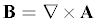(1)

between magnetic flux density, B and magnetic vector potential, A According to Maxwell’s equations we have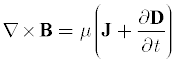(2)

where μ is the space permeability, J is the electric current density, and D is the electric displacement field. If we take curl of both sides of (1) and use (2), we have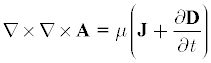(3)

With the use of identity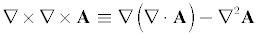(4)

(3) can be written as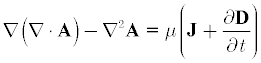(5)

We assume Coulomb gauge,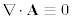, for vector potential to simplify (5) as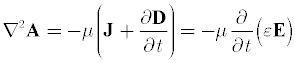(6)

where ε is the electric permittivity. The second equation is written since there is no free electric current density in space. We also eliminated electric displacement in favor of electric field, E We can write electric field in its general form as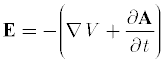(7)

Where V is the electric potential. Considering sinusoidal steady state, we can write (7) as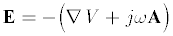(8)

Combining (8) and (6) we have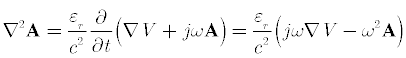(9)

According to Gauss’s law, when there is no free electric charge in space, ρ = 0 , electric field satisfies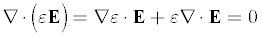(10)

Replacing E with its value from (8) and using Coulomb gauge we obtain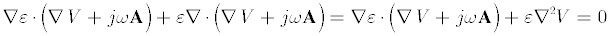(11)

We can write (11) in a different form as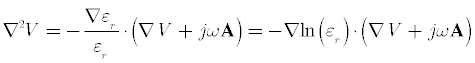(12)

An example of a slab PC that we want to calculate its modes is shown in Figure 1. As can be seen, permittivity of space and its logarithm can be written as Fourier series with coefficients depend on z component,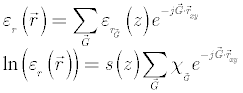(13)

Here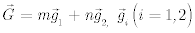are primitive reciprocal lattice vectors of a two-dimensional crystal with the same permittivity pattern, where m, n are integers and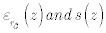are defined as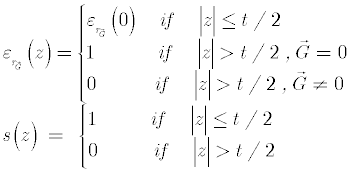(14)

According to Bloch theorem, magnetic vector potential and electric potential in this problem are of the form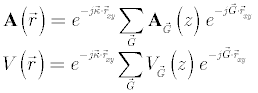(15)

where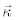is the Bloch wave vector that is supposed to be in the plane of slab, i.e.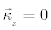. Replacing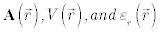in (9) with their values from (15) and (13) and assuming, c=1, according to the conventional normalized angular frequency scheme, we obtain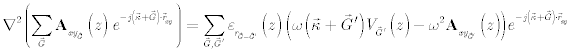(16)

for the in-plane, xy , component of vector potential. We can change (16) from differential to integral form, by using Laplace operator Green’s function ,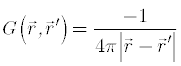(17)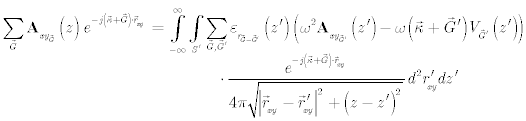(18)

With the help of identity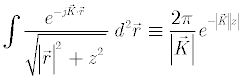(19)

(18) simplifies to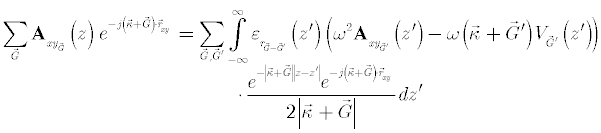(20)

Again because of orthogonality of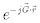for all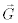in reciprocal lattice, we can further simplify (20) as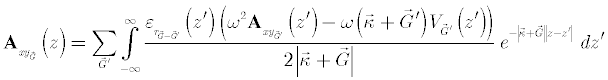(21)

If we go the same process for the z component of (9) we obtain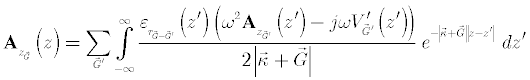(22)

where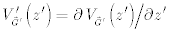. The last equation we need to be able to solve our slab PC problem is obtained by replacing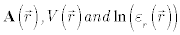in (12) with their values from (15) and (13). Again by using Laplace operator Green’s function and doing some simplification we reach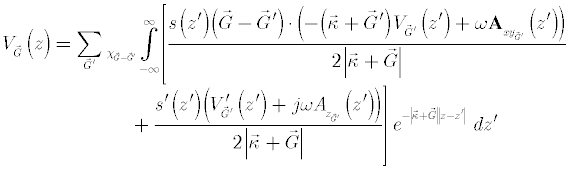(23)

We now take dot product of both sides of (21) by the unit vector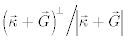to obtain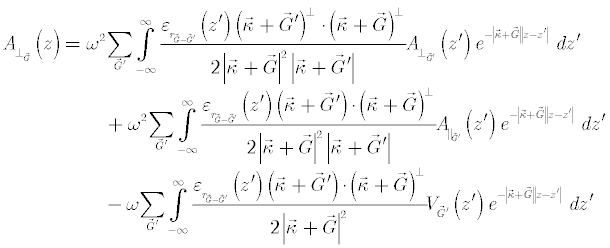(24)

where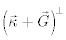is perpendicular to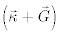but with the same magnitude.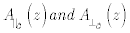are projections of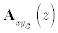onto the direction of wave propagation,, and direction of perpendicular to wave propagation and slab normal respectively. We can write (24) in matrix form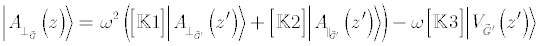(25)

where elements of matrices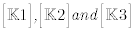are integral operators as follows,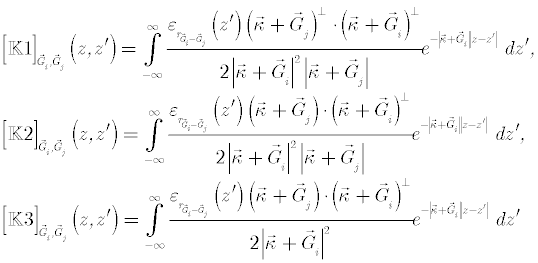(26)

We can now take dot product of both sides of (21) by the unit vector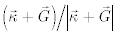to obtain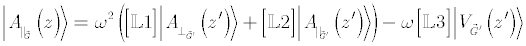(27)

where elements of matrices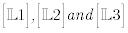are integral operators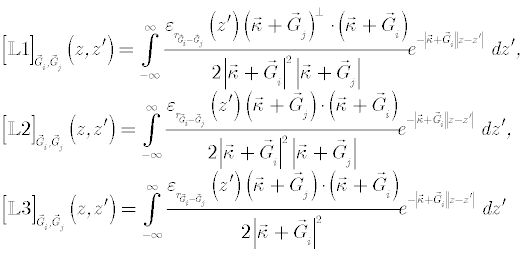(28)

We can also write (23) in matrix form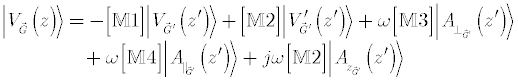(29)

where elements of matrices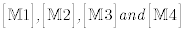are integral operators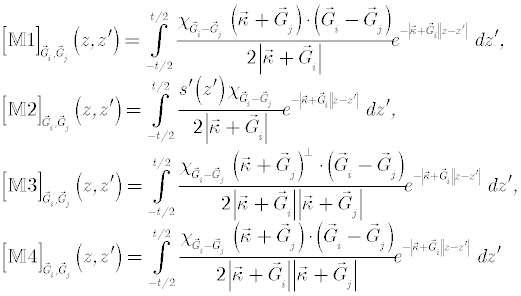(30)

If we can expand even and odd functions of z component in our equations respectively by cosine or sine series within a distance around the slab, then we can easily write a linear equation which relates derivative of a function by itself. For instance we can write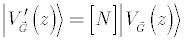(31)

where [N] is a block diagonal matrix like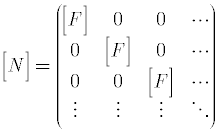(32)

where [F] equals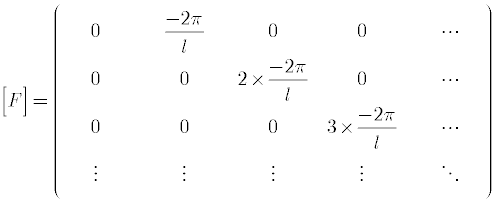(33)

and l is the distance around the slab which we have written the cosine and sine series in it. Combining (29) and (31) we can write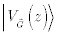as a function of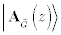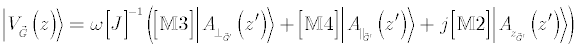(34)

Where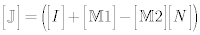. Replacingin (25) and (27) by its value from (34) we obtain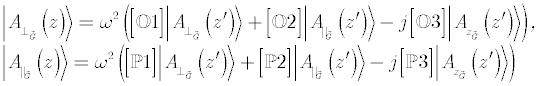(35)

where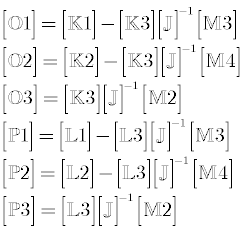(36)

Again we use Coulomb gauge to eliminate one ofcomponents in favor of the others. According to this gauge we have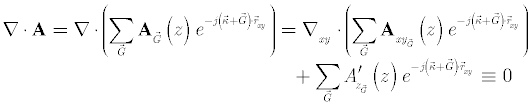(37)

Therefore we conclude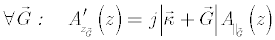(38)

We can write (38) in matrix form as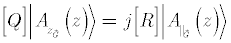(39)

provided that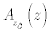can be approximated by cosine or sine series. Combining (35) and (39) we finally obtain the eigenvalue equation for the components of magnetic vector potential as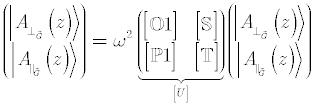(40)

where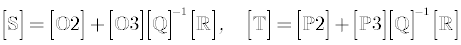(41)

We can calculate the normalized angular frequency w as a function of Bloch wave vector,, using the equation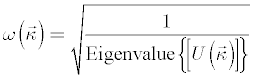(42)

Using (42) we have calculated TE-like band structure of the slab PC shown in Figure 1 with t = 0.6a . This band structure is shown in Figure 2 with Blue dots. For comparison result of FDTD method is also plotted with red circles.

Figure 1: Slab PC which its modes is calculated in this section. This crystal is formed by carving air cylinders inside a Silicon substrate with diameters equal 0 .7 lattice constant. Slab thickness is 0.6a .

Figure 2: TE-like band structure of the slab PC shown in Figure.1 with t = 0.6a . Blue dots are calculated from (42), red circles are results of FDTD, and magenta curves are obtained from three-dimensional tandard PWEM.

#### Performance Comparison

As mentioned in the above, the main advantage of our method over the common one is that its results converge faster as the size of the final matrix increase and this matrix is also prepared much more efficiently. Counter intuitively, calculation of the matrix operators in (40) and setup of the eigenvalue problem is much faster compared to the standard approach. The reason is as follows. Suppose that one would utilize N expansion terms along each direction. Then, the size of final eigenvalue problem in both standard and our new method would be identically N3 While the standard PWEM would require N3 individual calculation subroutines to setup the full coefficients matrix, our proposed scheme would require only N2 calculations given for a sub-matrix which apart from a scalar multiplication repeats to complete the full-sized matrix having the size N3 . Put in other words, while the standard PWEM is an algorithm with computational complexity O(N3) , this proposed algorithm has a computational complexity N2 . It is exactly for this reason that in practice our scheme turns out to be much more efficient and stable compared to the standard PWEM. To illustrate this we have plotted the first mode frequency of the slab PC of previous section at the high-symmetry point M(1) against the time it took to calculate it using our method, the standard PWEM, and FDTD in Figure 3. As it can be seen our method converges to the final result much faster within few seconds while for the FDTD and standard PWEM, one would need roughly at least half an hour to obtain a trustable result.

Figure 3: Comparison of our method with other ones. Frequency of the first mode of slab PC of previous section at M(1) in reciprocal lattice against the time it took to calculate it. Red line and circles are related to FDTD, blue line and dots are results of our method, and common method is shown with magenta line and squares.

#### Conclusion

In this paper we introduced a new method to find modes of a slab PC. In this method which is based on PWE, unlike the standard PWEM that change the slab PC structure to obtain a three-dimensional crystal, we consider the slab as a two-dimensional crystal but with Fourier series coefficients depend on slab normal component. We showed that with this method we can calculate slab PCs modes much faster and no super-computing is needed for it.

#### References

Select your language of interest to view the total content in your interested language

### Article Usage

• Total views: 12329
• [From(publication date):
December-2014 - Sep 17, 2019]
• Breakdown by view type
• HTML page views : 8519

## Post your commentCan't read the image? click here to refresh
###### Peer Reviewed Journals

Make the best use of Scientific Research and information from our 700 + peer reviewed, Open Access Journals

International Conferences 2019-20

Meet Inspiring Speakers and Experts at our 3000+ Global Annual Meetings

Top